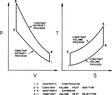# Entropy of Otto cycle

• Engineering
stephen0507
Homework Statement:
Determine s1 and s2 given P1= 90.0, P2= 2260.7, T1=283.1, and T2= 711.2
Relevant Equations:
P1= 90kpa
T1= 283.1K
v1/v2=10

P2=P1(v1/v2)^k= 90(10)^1.4= 2260.7kpa
T2=T1(v1/v2)^(k-1)= 283.1(10)^0.4= 711.2K
Hello, just wanted to ask regarding the otto cycle; if we were to find the entropy at the phase of isentropic compression and I was already able to derive the temperature 1 and 2 and the pressures at 1 and 2 and I also have the compression ratio of 10. How do I derive the entropy (s1 and s2)? given the fact that entropy at this phase is s1=s2.

#### Attachments

•1626334643448.png
6.6 KB · Views: 76

Mentor
Are you sure you are being asked to determine the absolute entropies of the two states? Of what value is that?

stephen0507
Are you sure you are being asked to determine the absolute entropies of the two states? Of what value is that?
They're asking for the datum of each phase it's more of an introductory class in thermodynamics so I guess they just want us to be familiar with the derivation of each point. So far, I was thinking of using the table of air properties to determine the entropies for each state since I have the temperatures and pressure of both phases 1 and 2. Would this be the right way to determine entropy (s) and internal energy (u)?

Mentor
They're asking for the datum of each phase it's more of an introductory class in thermodynamics so I guess they just want us to be familiar with the derivation of each point. So far, I was thinking of using the table of air properties to determine the entropies for each state since I have the temperatures and pressure of both phases 1 and 2. Would this be the right way to determine entropy (s) and internal energy (u)?
Is this course statistical thermodynamics?

Last edited:
•stephen0507
Mentor
Are you familiar with the equation for an ideal gas: $$dS=C_p\frac{dT}{T}-R\frac{dP}{P}=C_v\frac{dT}{T}+R\frac{dV}{V}$$

•stephen0507
stephen0507
yes! I actually wanted to ask about this. So I was able to determine entropy from points 1 and 2 using the ideal gas table for air since the otto cycle goes through an isentropic process is it safe to assume that s1=s2? so the entropy of air I got in phase 1 is the same entropy for the second phase? If so I need to get s3 and I was wondering if I could do this:

s3-s2= Cpln(T3/T2)-Rln(P3/P2)

and solve for s3

Mentor
yes! I actually wanted to ask about this. So I was able to determine entropy from points 1 and 2 using the ideal gas table for air since the otto cycle goes through an isentropic process is it safe to assume that s1=s2? so the entropy of air I got in phase 1 is the same entropy for the second phase?
This is OK, but please understand that the air table typically does not give the absolute entropy. It typically gives the entropy relative to some reference state like 25 C and 1 atm. Check the table to see what the use as the reference state of zero entropy.
If so I need to get s3 and I was wondering if I could do this:

s3-s2= Cpln(T3/T2)-Rln(P3/P2)

and solve for s3
Sure.

•stephen0507
stephen0507
This is OK, but please understand that the air table typically does not give the absolute entropy. It typically gives the entropy relative to some reference state like 25 C and 1 atm. Check the table to see what the use as the reference state of zero entropy.
ok yes understood I have noticed this in different references too, Ill take note of this thank you so much,

stephen0507
Sure.
Just to confirm I can use the equation that I set up to solve for entropy at 3 correct?

Mentor
Just to confirm I can use the equation that I set up to solve for entropy at 3 correct?
Yes.

•stephen0507
stephen0507
Thank you so much, you helped me with this I will keep note of everything you mentioned. Thanks!